# 开发者实战 | 自训练 YOLOv5 模型使用 OpenVINO™ 优化并部署在英特尔开发套件

09/18 17:001.1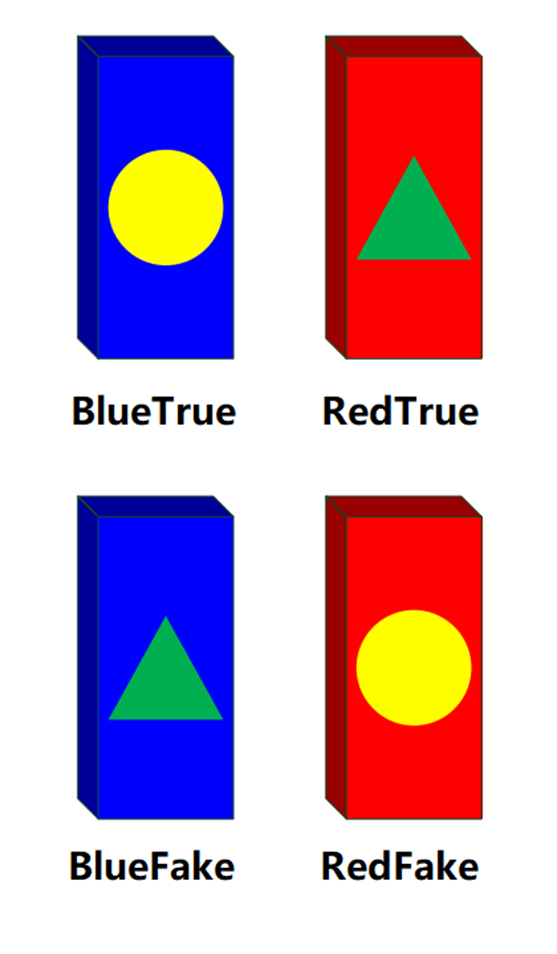1.2

YOLOv5 以及目标检测

YOLO (You Only Look Once) 是目标检测模型，目标检测是计算机视觉中一种重要的任务，目的是在一张图片中找出特定的物体，同时要求识别物体的种类和位置。在此之前的文章中 Pytorch 模型是用于图像分类，只要求识别画面中物体的种类。具体的区别通过图 1.2.1 直观可知。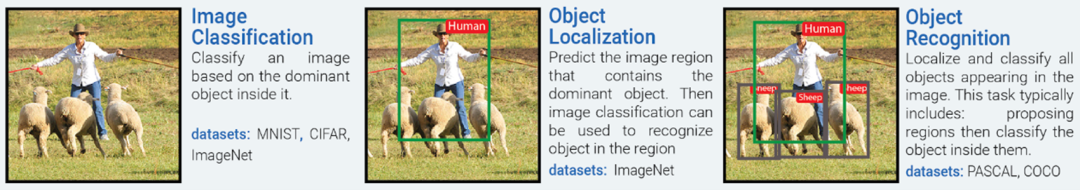01

Labelimg 是一款数据标注软件，支持输出包括 yolo, PascalVOC 等格式标注数据，这里我们选择 yolo 格式即可。

pip install labelimg -i https://mirror.baidu.com/pypi/simple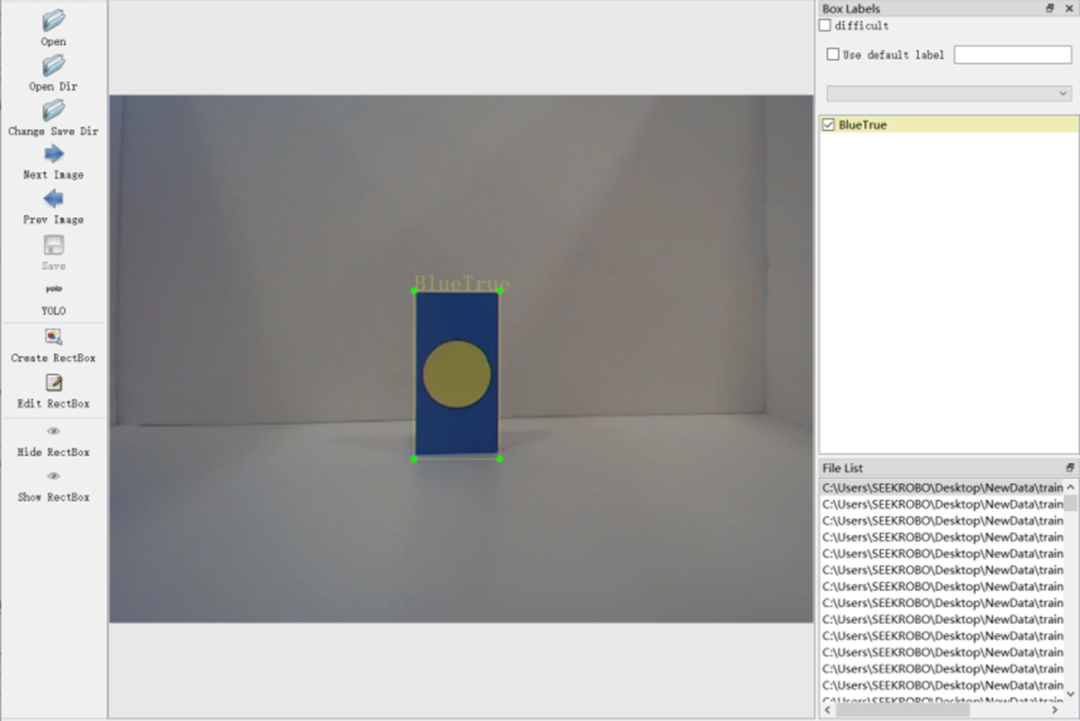02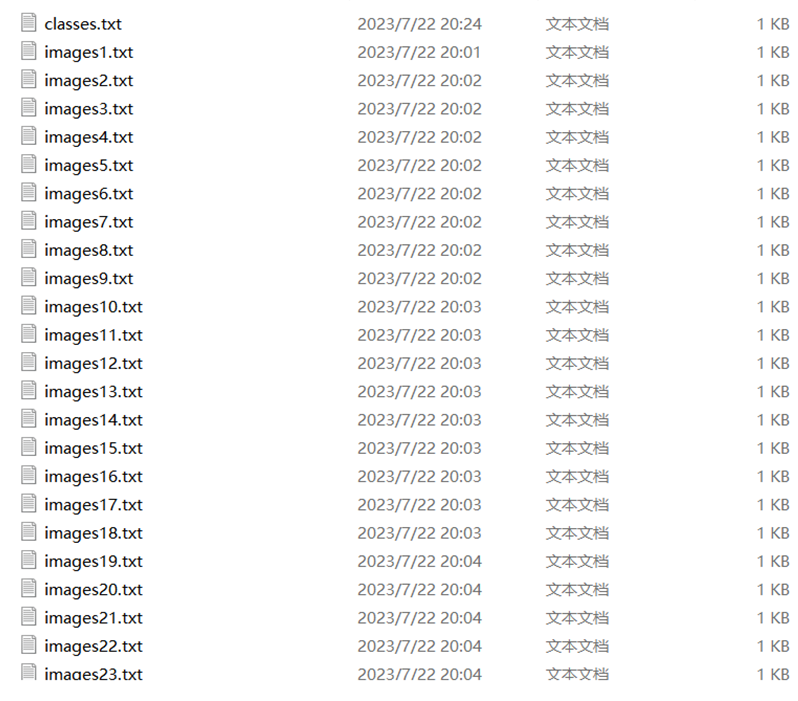03

1.3

1.3.1

import openvino.runtime as ovimport cv2import numpy as npimport openvino.preprocess as op

PreProcess API 介绍：

OpenVINO™ PreProcess 是 OpenVINO™ Python API 大家庭的一员，主要是提供了一个 OpenVINO™ Runtime 原生用于数据预处理的 API 函数库，在不用 PreProcess 时，开发者需要用第三方库例如 OpenCV 来对其进行预处理，但是 OpenCV 作为一个开源的、广泛的功能库，数据预处理只能加载到 CPU 去进行实现，这无疑是增加对 CPU 资源的开销，并且之后需要将处理后数据再次返还到 iGPU 等计算设备进行推理。而 PreProcess 提供了一种方式，使得预处理也能直接集成到模型执行图中去，整个模型工作流程都在 iGPU 上流转，这样无需依赖 CPU，能提高执行效率。

1.3.2

def Init():    global core    global model    global compiled_modelglobal infer_request#核心创建core = ov.Core() #读取用YOLOv5模型转换而来的IR模型model = core.read_model("best2.xml", "best2.bin") #运用PPP(PrePostProcessor)对模型进行预处理Premodel = op.PrePostProcessor(model)Premodel.input().tensor().set_element_type(ov.Type.u8).set_layout(ov.Layout("NHWC")).set_color_format(op.ColorFormat.BGR)Premodel.input().preprocess().convert_element_type(ov.Type.f32).convert_color(op.ColorFormat.RGB).scale(        [255., 255., 255.])    Premodel.input().model().set_layout(ov.Layout("NCHW"))    Premodel.output(0).tensor().set_element_type(ov.Type.f32)    model = Premodel.build()    compiled_model = core.compile_model(model, "CPU") #加载模型，可用CPU or GPU    infer_request = compiled_model.create_infer_request() #生成推理

1.3.3

def resizeimg(image, new_shape):old_size = image.shape[:2]#记录新形状和原生图像矩形形状的比率    ratio = float(new_shape[-1] / max(old_size))     new_size = tuple([int(x * ratio) for x in old_size])    image = cv2.resize(image, (new_size, new_size))    delta_w = new_shape - new_size    delta_h = new_shape - new_sizecolor = [100, 100, 100]new_im = cv2.copyMakeBorder(image, 0, delta_h, 0, delta_w, cv2.BORDER_CONSTANT, value=color)    #增广操作    return new_im, delta_w, delta_h

1.3.4

#************************************##               推理主程序             #def main(img,infer_request):    push =[]    img_re,dw,dh = resizeimg(img,(640,640)) #尺寸处理    input_tensor = np.expand_dims(img_re, 0) #获得输入张量    infer_request.infer({0: input_tensor}) #输入到推理引擎    output = infer_request.get_output_tensor(0) #获得推理结果    detections = output.data #获得检测数据    boxes = []    class_ids = []    confidences = []    for prediction in detections:        confidence = prediction.item() #获取置信度        if confidence >= 0.6: #初步过滤，过滤掉绝大多数的无效数据            classes_scores = prediction[5:]            _, _, _, max_indx = cv2.minMaxLoc(classes_scores)            class_id = max_indx            if (classes_scores[class_id] > .25):                confidences.append(confidence)                class_ids.append(class_id)                x, y, w, h = prediction.item(), prediction.item(), prediction.item(), prediction.item() #获取有效信息                xmin = x - (w / 2) #由于NMSBoxes缘故，需要从中心点得到左上角点                ymin = y - (h / 2)                box = np.array([xmin, ymin, w, h]) #记录数据                boxes.append(box)    indexes = cv2.dnn.NMSBoxes(boxes, confidences, 0.5, 0.5) #NMS筛选    detections = []    for i in indexes:        j = i.item()        detections.append({"class_index": class_ids[j], "confidence": confidences[j], "box": boxes[j]}) #储存获取的目标名称和框选位    for detection in detections:        box = detection["box"]        classId = detection["class_index"]        confidence = detection["confidence"]if(confidence<0.88): #再次过滤            continue        else :            push.append(classId)        rx = img.shape / (img_re.shape - dw)        ry = img.shape / (img_re.shape - dh)        img_re = cv2.rectangle(img_re, (int(box), int(box)), (int(box + box), int(box + box)), (0, 255, 0), 3)        box = rx * box #恢复原尺寸box，如果尺寸不变可以忽略        box = box *ry        box = rx * box        box = box *ry        xmax = box + box        ymax = box + box        img = cv2.rectangle(img, (int(box), int(box)), (int(xmax), int(ymax)), (0, 255, 0), 3) #绘制物体框        img = cv2.rectangle(img, (int(box), int(box) - 20), (int(xmax), int(box)), (0, 255, 0), cv2.FILLED) #绘制目标名称底色填充矩形        img = cv2.putText(img, str(label[classId])+'  '+str(int(confidence*100))+'%', (int(box), int(box) - 5), cv2.FONT_HERSHEY_SIMPLEX, 0.5, (0, 0, 0))  #绘制目标名称    cv2.imshow("d", img_re)    cv2.imshow('w',img)    cv2.waitKey(0)

#********************主程序***********************#def MainToSolve(infer):    img = cv2.imread("boundtest.jpg")  #如果需要实时，只需要将输入img变成从摄像机抓取的帧画面main(img,infer)#从这里开始，初始化以及推理Init()MainToSolve(infer_request)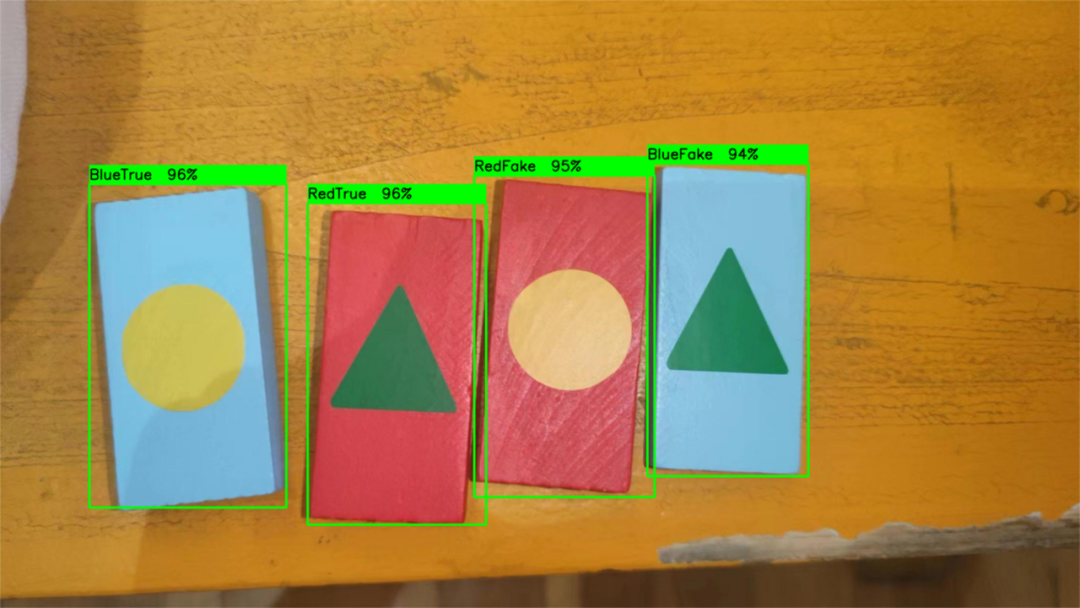1.4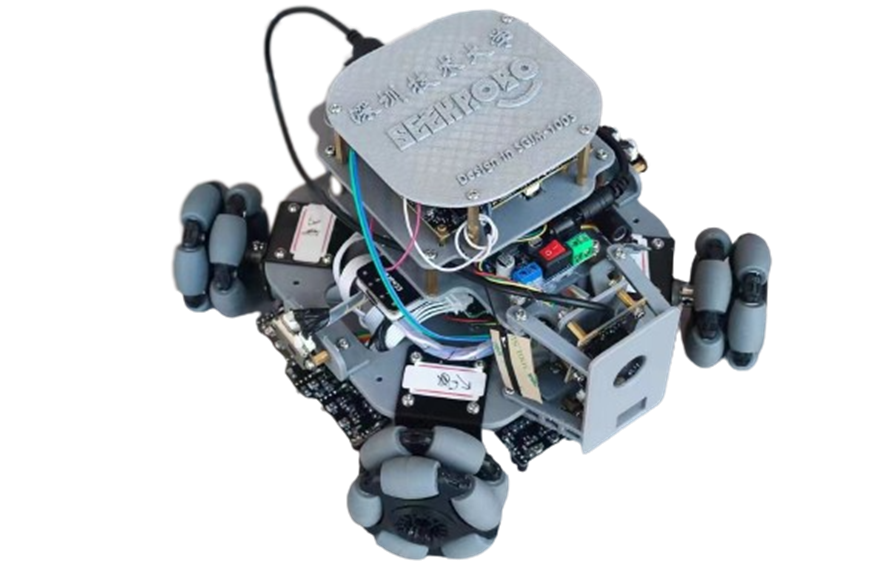1.5

OpenVINO™ 简单易上手，提供了健全的文档和 OpenVINO™ Notebooks 范例，帮助开发者专注在自身应用的实现和算法搭建。

OpenVINO™

--END--



你也许想了解(点击蓝字查看)⬇️

➡️ 基于 ChatGLM2 和 OpenVINO™ 打造中文聊天助手

➡️ 基于 Llama2 和 OpenVINO™ 打造聊天机器人

➡️ OpenVINO™ DevCon 2023重磅回归！英特尔以创新产品激发开发者无限潜能

➡️ 5周年更新 | OpenVINO™  2023.0，让AI部署和加速更容易

➡️ OpenVINO™5周年重头戏！2023.0版本持续升级AI部署和加速性能

➡️ OpenVINO™2023.0实战 | 在 LabVIEW 中部署 YOLOv8 目标检测模型

➡️ 开发者实战系列资源包来啦！

➡️

以AI作画，祝她节日快乐；简单三步，OpenVINO™ 助你轻松体验AIGC

➡️

还不知道如何用OpenVINO™作画？点击了解教程。

➡️

➡️

使用OpenVINO 在“端—边—云”快速实现高性能人工智能推理





扫描下方二维码立即体验
OpenVINO™ 工具套件 2023.0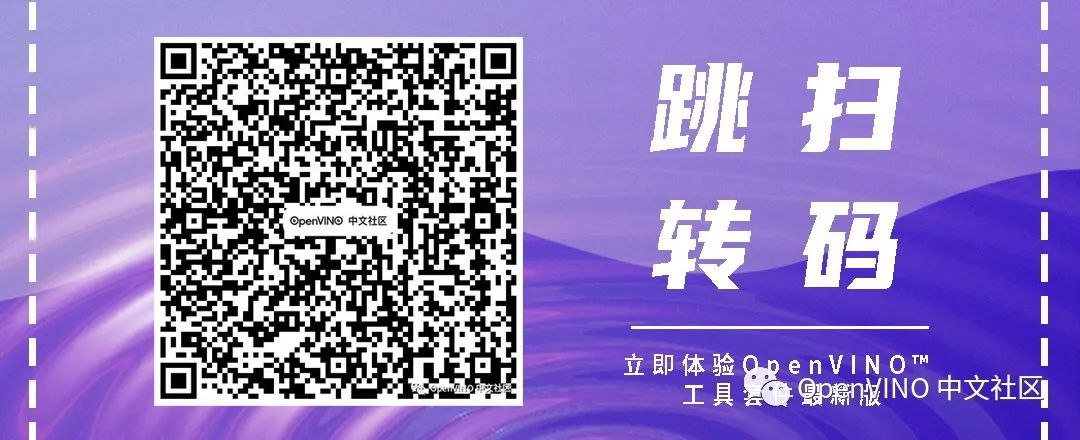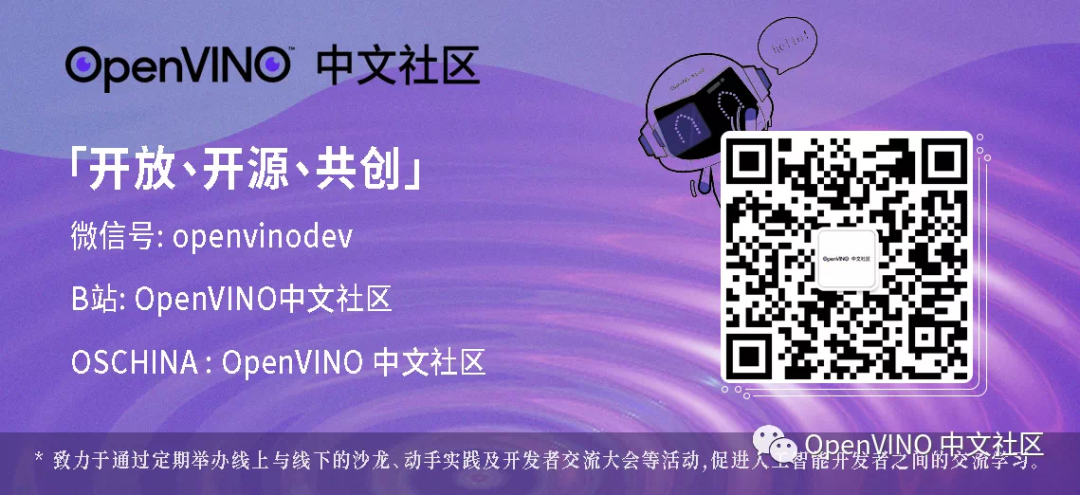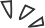0 评论
0 收藏
0